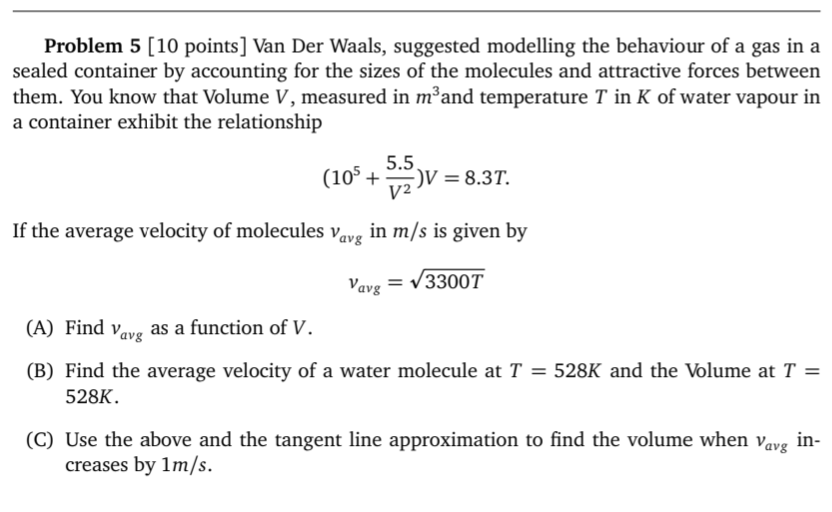# Question ​​​​​​​ Problem 5 [10 points] Van Der Waals, suggested modelling the behaviour of a gas in a sealed container by accounting for the sizes of the molecules and attractive forces between them. You know that Volume $$V$$, measured in $$m^{3}$$ and temperature $$T$$ in $$K$$ of water vapour in a container exhibit the relationship $\left(10^{5}+\frac{5.5}{V^{2}}\right) V=8.3 T$ If the average velocity of molecules $$v_{a v g}$$ in $$\mathrm{m} / \mathrm{s}$$ is given by $v_{a v g}=\sqrt{3300 T}$ (A) Find $$v_{a v g}$$ as a function of $$V$$. (B) Find the average velocity of a water molecule at $$T=528 \mathrm{~K}$$ and the Volume at $$T=$$ $$528 K$$ (C) Use the above and the tangent line approximation to find the volume when $$v_{\text {avg }}$$ increases by $$1 \mathrm{~m} / \mathrm{s}$$.​​​​​​​
Transcribed Image Text: Problem 5 [10 points] Van Der Waals, suggested modelling the behaviour of a gas in a sealed container by accounting for the sizes of the molecules and attractive forces between them. You know that Volume $$V$$, measured in $$m^{3}$$ and temperature $$T$$ in $$K$$ of water vapour in a container exhibit the relationship $\left(10^{5}+\frac{5.5}{V^{2}}\right) V=8.3 T$ If the average velocity of molecules $$v_{a v g}$$ in $$\mathrm{m} / \mathrm{s}$$ is given by $v_{a v g}=\sqrt{3300 T}$ (A) Find $$v_{a v g}$$ as a function of $$V$$. (B) Find the average velocity of a water molecule at $$T=528 \mathrm{~K}$$ and the Volume at $$T=$$ $$528 K$$ (C) Use the above and the tangent line approximation to find the volume when $$v_{\text {avg }}$$ increases by $$1 \mathrm{~m} / \mathrm{s}$$.
Transcribed Image Text: Problem 5 [10 points] Van Der Waals, suggested modelling the behaviour of a gas in a sealed container by accounting for the sizes of the molecules and attractive forces between them. You know that Volume $$V$$, measured in $$m^{3}$$ and temperature $$T$$ in $$K$$ of water vapour in a container exhibit the relationship $\left(10^{5}+\frac{5.5}{V^{2}}\right) V=8.3 T$ If the average velocity of molecules $$v_{a v g}$$ in $$\mathrm{m} / \mathrm{s}$$ is given by $v_{a v g}=\sqrt{3300 T}$ (A) Find $$v_{a v g}$$ as a function of $$V$$. (B) Find the average velocity of a water molecule at $$T=528 \mathrm{~K}$$ and the Volume at $$T=$$ $$528 K$$ (C) Use the above and the tangent line approximation to find the volume when $$v_{\text {avg }}$$ increases by $$1 \mathrm{~m} / \mathrm{s}$$.&#12304;General guidance&#12305;The answer provided below has been developed in a clear step by step manner.Step1/6(A) Using equation (A), we have:$$\mathrm{{v}_{{a}}{v}{g}=\sqrt{{{3{,}300}{T}}}}$$ ExplanationWe want to express this in terms of V, so we need to eliminate T using the given relationship between V and T:PV = nRTwhere P is the pressure, n is the number of moles of gas, and R is the ideal gas constant. Explanation:Please refer to solution in this step.Step2/6For water vapour, we can use the Van der Waals equation: $$\mathrm{{\left({P}+{a}{\left(\frac{{n}}{{V}}\right)}^{{2}}\right)}{\left({V}-{n}{b}\right)}={n}{R}{T}}$$where a and b are constants that depend on the properties of the gas. Rearranging, we get:P = (nRT)/(V - nb) - a(n/V)^2. Substituting this expression for P into the ideal gas law, we get:$$\mathrm{\frac{{{n}{R}{T}}}{{{V}-{n}{b}}}-{a}{\left(\frac{{n}}{{V}}\right)}^{{2}}={R}\frac{{T}}{{V}}}$$Simplifying, we get:$$\mathrm{{n}{R}{T}={\left({R}\frac{{T}}{{V}}\right)}{\left({V}-{n}{b}\right)}+{a}{\left(\frac{{n}}{{V}}\right)}^{{2}}{\left({V}-{n}{b}\right)}}$$Explanation:Please refer to solution in this step.Step3/6Expanding and canceling terms, we get:nRT = RT - nRTb/V + a(n^2/V^2)(V - nb)Solving for T, we get:T = (RT)/(nR - b/V) - (a/V)(n/V). Substituting this expression for T into equation (A), we get:$$\mathrm{{v}_{{a}}{v}{g}=\sqrt{{{3{,}300}{\left[\frac{{{R}{T}}}{{{n}{R}-\frac{{b}}{{V}}}}-{\left(\frac{{a}}{{V}}\right)}{\left(\frac{{n}}{{V}}\right)}\right]}}}}$$ Simplifying, we get:$$\mathrm{{v}_{{a}}{v}{g}=\sqrt{{{\left({3{,}300}\frac{{R}}{{n}}\right)}{\left(\frac{{T}}{{{1}-\frac{{b}}{{V}}}}\right)}-{\left({3{,}300}\frac{{a}}{{n}}{V}\right)}}}}$$Explanation:Please refer to solution in this step.Step4/6( ... See the full answer